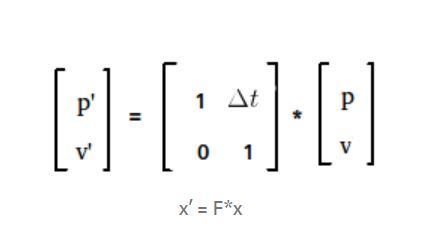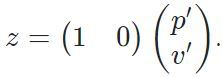# Overview of Kalman Filter for Self-Driving Car

A Kalman Filter is an optimal estimation algorithm. It can help us predict/estimate the position of an object when we are in a state of doubt due to different limitations such as accuracy or physical constraints which we will discuss in a short while.

Application of Kalman filter:
Kalman filters are used when –

• Variable of interest that can only be measured indirectly.
• Measurements are available from various sensors but might be subject to noise i.e Sensor Fusion.

Let’s dig deep into each of the uses one by one.

1. Indirect measurement:
To measure the Variable of interest(variable under consideration) that can only be measured indirectly. This type of variable is called the state estimated variable. Let’s understand it with an example.
Example:
Let’s say that you want to know how happy your dog Jacky is. Thus your variable of interest, y is Jacky’s happiness. Now the only way to measure jacky’s happiness is to measure it indirectly as happiness is not a physical state that can be measured directly. You can choose to see Jacky waves his tail and predict whether he is happy or not. You also might have a whole different approach to give you an idea or estimate how happy Jacky is. This unique idea is the Kalman Filter. And that’s what I meant when I said that Kalman filter is an optimal estimation algorithm.
2. Sensor Fusion:
Now you have the intuition of what this filter exactly is. Kalman Filter combines the measurement and the prediction to find the optimal estimate of the car’s position.
Example:
Suppose you have a remote-controlled car and its running at a speed of 1 m/s. let’s say that after 1 second you need to predict the exact position of the car what will be your prediction??
Well if you know some basic time, distance and speed formula you will be correctly able to say 1 meter ahead of the current position. But how accurate is this model??
There are always some variations from the ideal scenario and that deviation is the cause of the error. Now to minimize the error in the state prediction, the measurement from a sensor is taken. The sensor measurement has some error as well. So now we have two probability distributions(the sensor and the prediction as they are not always a number but the probability distribution function(pdf)) that are supposed to tell the location of the car. Once we combine these two gaussian curve we can get a whole new gaussian that has far less variance.
Example:
Suppose you have a friend(Sensor 2) who is good at maths and not that great in physics and you(Sensor 1) are good at physics but not so much in maths. Now on the day of the examination, when your target is getting a good result, you and your friend come together in order to excel in both the subjects. You both collaborate to minimize the error and maximize the result(output).
Example:
It’s just like when you need to find out about an incident, you ask different individuals about it and after listening to all their stories, you make your own which, you seem is far accurate than any of the individual stories. See its always related to our daily lives and we can always make a connection with what we have already experienced.

Code: Python implementation of the 1-D Kalman filter

 `def` `update(mean1, var1, mean2, var2): ` `    ``new_mean ``=` `float``(var2 ``*` `mean1 ``+` `var1 ``*` `mean2) ``/` `(var1 ``+` `var2) ` `    ``new_var ``=` `1.``/``(``1.``/``var1 ``+` `1.``/``var2) ` `    ``return` `[new_mean, new_var] ` ` `  `def` `predict(mean1, var1, mean2, var2): ` `    ``new_mean ``=` `mean1 ``+` `mean2 ` `    ``new_var ``=` `var1 ``+` `var2 ` `    ``return` `[new_mean, new_var] ` ` `  `measurements ``=` `[``5.``, ``6.``, ``7.``, ``9.``, ``10.``] ` `motion ``=` `[``1.``, ``1.``, ``2.``, ``1.``, ``1.``] ` `measurement_sig ``=` `4.` `motion_sig ``=` `2.` `mu ``=` `0.` `sig ``=` `10000.` ` `  `# print out ONLY the final values of the mean ` `although ``for` `a better understanding you may choose to  ` `# and the variance in a list [mu, sig].  ` ` `  `for` `measurement, motion ``in` `zip``(measurements, motion): ` `    ``mu, sig ``=` `update(measurement, measurement_sig, mu, sig) ` `    ``mu, sig ``=` `predict(motion, motion_sig, mu, sig) ` `print``([mu, sig]) `

Explanation:
As we have discussed there are two major steps in the complete process first Update step and then prediction step. These two steps are looped over and over to estimate the exact position of the robot.

The prediction step :
New position p’ can be calculated using the formula

p’ = p + v * dt

where p is the previous position, v is the velocity and dt is the time-step.
new velocity v’ will be the same as the previous velocity as its constant(assumption). This in equation can be given as
v’ = v

Now to write this complete thing in a single matrix.Prediction Step

The update step :
The filter you just implemented is in python and that too in 1-D. Mostly we deal with more than one dimension and the language changes for the same. So let’s implement a Kalman filter in C++.

Requiremnt:
Eigen library
You will need the Eigen library, especially the Dense class in order to work with the linear algebra required in the process. Download the library and paste it in the folder containing the code files, in case you don’t know how libraries work in C++. Also go through the official documentation for a better understanding of how to use its functionality. I have to admit the way they have explained in the documentation is amazing and better than any tutorial you can ask for.

Now implementing the same prediction and update function in c++ using this new weapon(library) we have found to deal with the algebra part in the process.
Prediction Step:

x’=F*x + B*u + v
P’ = F * P * F.transpose() + Q

Here B.u becomes zero as this is used to incorporate changes related to friction or any other force that is hard to calculate. v is the process noise which determines random noise that can be present in the system as a whole.

 `void` `KalmanFilter::Predict() ` `{ ` `    ``x = F * x; ` `    ``MatrixXd Ft = F.transpose(); ` `    ``P = F * P * Ft + Q; ` `} `

With that, we were able to calculate the predicted value of X and the covariance matrix P.

Update Step:

z = H * x + w

where w represents sensor measurement noise.

So for lidar, the measurement function looks like this:

z = p’

It also can be represented in a matrix form:Representation in a Matrix form

In this step, we use the latest measurements to update our estimate and our uncertainty.

H is the measurement function that maps the state to the measurement and helps in calculating the Error (y) by comparing the measurement(z) with our prediction(H*x).

y= z – H*x

The error is mapped into a matrix S, which is obtained by projecting the System Uncertainty(P) into the measurement space using the measurement function(H) + Matrix R that characters measurement Noise.
S = H * P * H.transpose() + R

This is then mapped into the variable called K. K is the Kalman gain and decides whether the measurement taken needs to be given more weight or the prediction according to the previous data and its uncertainty.
K = P*H.transpose() * S.inverse()

And then finally we update our estimate(x) and our uncertainty(P) using this equation using Kalman gain.

x = x + (K * y)
P = (I – K * H) * P

Here I is an identity matrix.

 `void` `KalmanFilter::Update(``const` `VectorXd& z) ` `{ ` `    ``VectorXd z_pred = H * x; ` `    ``VectorXd y = z - z_pred; ` `    ``MatrixXd Ht = H.transpose(); ` `    ``MatrixXd S = H * P * Ht + R; ` `    ``MatrixXd Si = S.inverse(); ` `    ``MatrixXd PHt = P * Ht; ` `    ``MatrixXd K = PHt * Si; ` ` `  `    ``// new estimate ` `    ``x = x + (K * y); ` `    ``long` `x_size = x_.size(); ` `    ``MatrixXd I = MatrixXd::Identity(x_size, x_size); ` `    ``P = (I - K * H) * P; ` `} `

Code:

 `// create a 4D state vector, we don't know yet the values of the x state ` `x = VectorXd(4); ` ` `  `// state covariance matrix P ` `P = MatrixXd(4, 4); ` `P << 1, 0, 0, 0, ` `    ``0, 1, 0, 0, ` `    ``0, 0, 1000, 0, ` `    ``0, 0, 0, 1000; ` ` `  `// measurement covariance ` `R = MatrixXd(2, 2); ` `R << 0.0225, 0, ` `    ``0, 0.0225; ` ` `  `// measurement matrix ` `H = MatrixXd(2, 4); ` `H << 1, 0, 0, 0, ` `    ``0, 1, 0, 0; ` ` `  `// the initial transition matrix F ` `F = MatrixXd(4, 4); ` `F << 1, 0, 1, 0, ` `    ``0, 1, 0, 1, ` `    ``0, 0, 1, 0, ` `    ``0, 0, 0, 1; ` ` `  `// set the acceleration noise components ` `noise_ax = 5; ` `noise_ay = 5; `

The reason why I didn’t initialize the matrices at first is that that is not the main part while we are writing a Kalman filter. First, we should know how the two main function works. then come the initialization and other stuff.

Some drawbacks :

• We are making this Kalman filter model in order to deal with lidar data that can be dealt with a linear function to predict. Well, we don’t use the only Lidar in a Self-driving car. we also use Radar and to use it we need to make some adjustments in the same code but for starters, this is perfect.
• We are assuming that the vehicle traced is moving at a constant velocity which is a big assumption thus we will be using a CTRV model which stands for Constant turn rate and velocity magnitude model and while dealing with that we will see a whole new approach to complete the task called the Unscented Kalman filter.

• My Personal Notes arrow_drop_upCheck out this Author's contributed articles.

If you like GeeksforGeeks and would like to contribute, you can also write an article using contribute.geeksforgeeks.org or mail your article to contribute@geeksforgeeks.org. See your article appearing on the GeeksforGeeks main page and help other Geeks.

Please Improve this article if you find anything incorrect by clicking on the "Improve Article" button below.

Article Tags :

Be the First to upvote.

Please write to us at contribute@geeksforgeeks.org to report any issue with the above content.